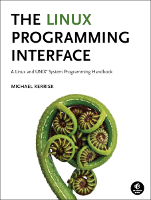This is fileio/t_readv.c (Listing 5-2, page 101), an example from the book, The Linux Programming Interface.

This page shows the "distribution" or "book" version of the file (why are there two versions?), or the differences between the two versions. You can switch between the views using the tabs below.

In the listing below, the names of Linux system calls and C library functions are hyperlinked to manual pages from the Linux man-pages project, and the names of functions implemented in the book are hyperlinked to the implementations of those functions.## Function list (Bold in this list means a function is not static)

```/* t_readv.c

Demonstrate the use of the readv() system call to perform "gather I/O".

(This program is merely intended to provide a code snippet for the book;
unless you construct a suitably formatted input file, it can't be
usefully executed.)
*/
#include <sys/stat.h>
#include <sys/uio.h>
#include <fcntl.h>
#include "tlpi_hdr.h"
```
```int
main(int argc, char *argv[])
{
int fd;
struct iovec iov;
struct stat myStruct;       /* First buffer */
int x;                      /* Second buffer */
#define STR_SIZE 100
char str[STR_SIZE];         /* Third buffer */

if (argc != 2 || strcmp(argv, "--help") == 0)
usageErr("%s file\n", argv);

fd = open(argv, O_RDONLY);
if (fd == -1)
errExit("open");

totRequired = 0;

iov.iov_base = &myStruct;
iov.iov_len = sizeof(struct stat);
totRequired += iov.iov_len;

iov.iov_base = &x;
iov.iov_len = sizeof(x);
totRequired += iov.iov_len;

iov.iov_base = str;
iov.iov_len = STR_SIZE;
totRequired += iov.iov_len;